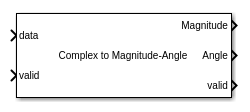# Complex to Magnitude-Angle

Compute magnitude and phase angle of complex signal using CORDIC algorithm

•Libraries:
DSP HDL Toolbox / Math Functions

## Description

The Complex to Magnitude-Angle block computes the magnitude and phase angle of a complex signal and provides hardware-friendly control signals. To achieve an efficient HDL implementation, the block uses a pipelined Coordinate Rotation Digital Computer (CORDIC) algorithm.

You can use this block to implement operations such as `atan2` in hardware.

## Ports

### Input

expand all

Complex input signal, specified as a scalar, a column vector representing samples in time, or a row vector representing channels. Using vector input increases data throughput while using more hardware resources. The block implements the conversion logic in parallel for each element of the vector. The input vector can contain up to 64 elements.

The software supports `double` and `single` data types for simulation, but not for HDL code generation.

Data Types: `single` | `double` | `int8` | `int16` | `int32` | `int64` | `uint8` | `uint16` | `uint32` | `uint64` | `fixed point`
Complex Number Support: Yes

Control signal that indicates if the input data is valid. When valid is `1` (`true`), the block captures values from the input data port. When valid is `0` (`false`), the block ignores the values from the input data port.

Data Types: `Boolean`

### Output

expand all

Magnitude of the input signal, returned as a scalar, a column vector representing samples in time, or a row vector representing channels. The dimensions of this port match the dimensions of the input data port.

#### Dependencies

To enable this port, set the Output format parameter to `Magnitude and Angle` or `Magnitude`.

Data Types: `single` | `double` | `int8` | `int16` | `int32` | `int64` | `uint8` | `uint16` | `uint32` | `uint64` | `fixdt([],N,0)`

Angle of the input signal, returned as a scalar, a column vector representing samples in time, or a row vector representing channels. The dimensions of this port match the dimensions of the input data port.

#### Dependencies

To enable this port, set the Output format parameter to `Magnitude and Angle` or `Angle`.

Data Types: `single` | `double` | `int8` | `int16` | `int32` | `int64` | `uint8` | `uint16` | `uint32` | `uint64` | `fixdt([],N,0)`

Control signal that indicates if the data from the output data port is valid. When valid is `1` (`true`), the block returns valid data from the Magnitude and/or Angle ports. When valid is `0` (`false`), the values from the Magnitude and/or Angle ports are not valid.

Data Types: `Boolean`

## Parameters

expand all

• To set the number of iterations to input WL − 1, select `Auto`. If the input is of data type `double` or `single`, the number of iterations is set to 16, by default.

• To specify the number of iterations by using Number of iterations parameter, select `Property`.

The number of iterations must be less than or equal to input WL − 1. The latency of the block depends on the number of iterations performed. For information about latency, see Latency.

#### Dependencies

To enable this parameter, set Number of iterations source to `Property`.

Use this parameter to specify which output ports are enabled.

• To enable the Magnitude and Angle output ports, select `Magnitude and Angle` (default).

• To enable the Magnitude output port and disable the Angle output port, select `Magnitude`.

• To enable the Angle output port and disable the Magnitude output port, select `Angle`.

• To return the Angle output as a fixed-point value that normalizes the angles in the range [–1,1], select` Normalized`. For more information see Normalized Angle Format.

• To return the Angle output as a fixed-point value in the range [-π, π], select `Radians`. When using this block to implement the `atan2` function, set this parameter to `Radians`.

Select this parameter to multiply the Angle output by the inverse of the CORDIC gain factor. The block implements this gain factor with either CSD logic or a multiplier, according to the Scaling method parameter.

Note

If you clear this parameter and apply the CORDIC gain elsewhere in your design, you must exclude the π/4 term. Because the quadrant mapping algorithm replaces the first CORDIC iteration by mapping inputs onto the angle range [0, π/4], the initial rotation does not contribute a gain term. The gain factor is the product of `cos(atan(2-n))`, for n from 1 to Number of iterations – 1.

When you set this parameter to `CSD`, the block implements the CORDIC gain scaling by using a shift-and-add architecture for the multiply operation. This implementation uses no multiplier resources and may increase the length of the critical path in your design. When you select `Multipliers`, the block implements the CORDIC gain scaling with a multiplier and increases the latency of the block by four cycles.

#### Dependencies

To enable this parameter, select the Scale output parameter.

expand all

## Version History

Introduced in R2014b

expand all